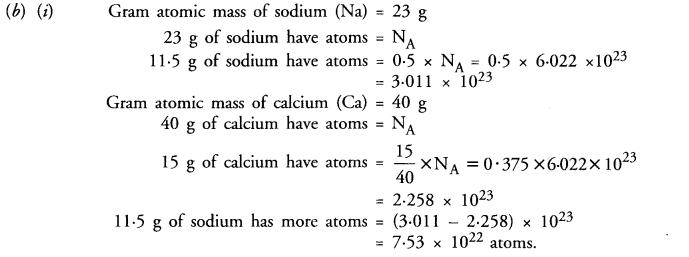## Online Education NCERT Solutions For Class 9 Science Chapter 3 Atoms and Molecules

These Solutions are part of Online Education NCERT Solutions for Class 9 Science. Here we have given NCERT Solutions for Class 9 Science Chapter 3 Atoms and Molecules. LearnInsta.com provides you the Free PDF download of NCERT Solutions for Class 9 Science (Chemistry) Chapter 3 – Atoms and Molecules solved by Expert Teachers as per NCERT (CBSE) Book guidelines. All Chapter 3 – Atoms and Molecules Exercise Questions with Solutions to help you to revise complete Syllabus and Score More marks.

More Resources

NCERT IN TEXT PROBLEMS

IN TEXT QUESTIONS

Question 1.
In a reaction, 5.3 g of sodium carbonate reacted with 6.0 g of ethanoic acid. The products were 2.2 g of carbon dioxide, 0.9 g of water and 8.2 g of sodium ethanoate. Show that these observations are in agreement with the law of conservation of mass. (CBSE 2012)
The chemical reaction leading to products is :
sodium carbonate + ethanoic acid ———–> sodium ethanoate + carbon dioxide + water.
Mass of reactants = (5.3 + 6.0) = 1.3 g
Mass of products = (8.2 + 2.2 + 0.9) = 11.3 g.
The reactants and products have same mass. This means that there was no loss of mass during the reaction. Therefore, the data is in agreement with law of conservation of mass.

Question 2.
Hydrogen and oxygen combine in the ratio of 1 : 8 by mass to form water. What mass of oxygen gas would be required to react with 3 g of hydrogen gas ?
According to available data,
Mass of oxygen combining with 1 g of hydrogen = 8 g.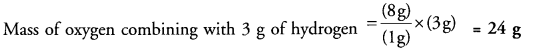Question 3:
Which postulate of Dalton’s Atomic theory is the basis of law of conservation of mass ? (CBSE 2012)
The law of conservation mass is based on the following postulate of Dalton’s Atomic theory.
“Atoms can neither be created nor destroyed during a physical change or a chemical reaction.”

Question 4.
Which postulate of Dalton’s Atomic theory can explain the law of definite proportions ?
The law of definite proportions is based on the following postulate of Dalton’s Atomic theory.
“All atoms of a particular element are identical in every respect. This means that they have same mass, same size and also same chemical properties.”

Question 5.
Define atomic mass unit.
Atomic mass unit may be defined as :
The mass of one-twelfth (1/12) of the mass of one atom of carbon taken as 12 u. It is represented as 1 u (unified mass).

Question 6.
Why is not possible to see an atom with naked eye ?
It is not possible to see an atom with naked eye because of its extremely small size. For example, the radius of an atom of hydrogen is of the order of 10-10 m. Actually an atom is regarded as a microscopic particle. These microscopic particles cannot be seen with naked eye.

Question 7.
Write down the formulae of :
(i) sodium oxide
(ii) aluminium chloride
(iii) sodium sulphide
(iv) magnesium hydroxide.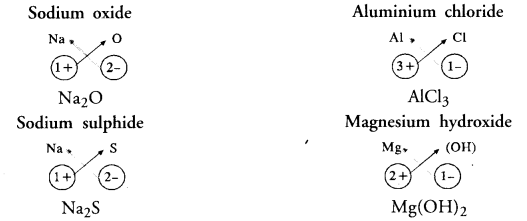Question 8.
Write the names of the compounds represented by the following formulae :
(i) Al2(SO4)3
(ii) CaCl2
(Hi) K2SO4
(iv) KNO3
(v) CaCO3.
(i) Aluminium sulphate
(ii) Calcium chloride
(iii) Potassium sulphate
(iv) Potassium nitrate
(v) Calcium carbonate

Question 9.
What is meant by the term chemical formula ?
Molecule represents a group of two or more atoms (same or different) chemically bonded to each other and held tightly by strong attractive forces. Molecules are represented in terms of symbols of constituting atoms and it is known as chemical formula.

Question 10.
How many atoms are present in
(i) H2S molecule
(ii) PO43- ion ?
(i) Three
(ii) Five.

Question 11.
Calculate the molecular masses of :
(i) H2
(ii) O2
(iii) Cl2
(iv) CO2
(v) CH4
(vi) C2H6
(vii) C2H4
(viii) NH3
(ix) CH3OH.
(i) Hydrogen (H2)
Molecular mass of H2 = 2 x Atomic mass of H = (2 x 1 u) = 2 u.
(ii) Oxygen (O2)
Molecular mass of O2 = 2 x Atomic mass of O = (2 x 16 u) = 32 u.
(iii) Chlorine (CI2)
Molecular mass of Cl2 = 2 x Atomic mass of Cl = (2 x 35’5 u) = 71 u.
(iv) Carbon dioxide (CO2)
Molecular mass of CO2 = (1 x Atomic mass of C) + (2 x Atomic mass of O)
= (1 x 12 u) + (2 x 16 u) = 12 u + 32 u = 44 u.
(v) Methane (CH4)
Molecular mass of CH4 = ( 1 x Atomic mass of C) + (4 x Atomic mass of H)
= ( 1 x 12 u) + (4 x 1 u) = 16 u.
(vi) Ethane (C2H6)
Molecular mass of C2H6 = (2 x Atomic mass of C) + (6 x Atomic mass of H)
= (2 x 12 u) + (6 x 1 u) = 30 u.
(vii) Ethylene (C2H4)
Molecular mass of C2H4 =(2 x Atomic mass of C) + (4 x Atomic mass of H)
= (2 x 12 u) + (4 x 1 u) = 28 u.
(viii) Ammonia (NH3)
Molecular mass of NH3 = (1 x Atomic mass ofN) + (3 x Atomic mass of H)
= (1 x 14 u) + ( 3 x 1 u) = 17 u.
(ix) Methyl alcohol (CH3OH)
Molecular mass of CH3OH = (1 x Atomic mass of C) + (4 x Atomic mass of H)
+ (1 x Atomic mass of O)
= (1 x 12 u) + (4 x 1 u) + (1 x 16 u) = 32 u.

Question 12.
Calculate the formula unit mass of :
(i) ZnO
(ii) Na2O
(iii) K2CO3.
Given : Atomic masses of Zn = 65 u, Na = 23 u, K = 39 u, C = 12 u and O = 16 u.
(i) Formula unit mass of ZnO (Zinc oxide)
= (1 x Atomic mass of Zn) +(1 x Atomic mass of O)
= (1 x 65 u) + (1 x 16 u) = 81 u.
(ii) Formula unit mass of Na2O (Sodium oxide)
= (2 x Atomic mass of Na) + (1 x Atomic mass of O)
= (2 x 23 u) + (1 x 16 u) = 62 u.
(iii) Formula unit mass of K2CO3 (Potassium carbonate).
= (2 x Atomic mass of K) + (1 x Atomic mass of C) + (3 x Atomic mass of O)
= (2 x 39 u) + (1 x 12 u) + (3 x 16 u) = 138 u

Question 13.
Calculate the number of moles of the following :
(i) 52 g of He
(ii) 12.044 x 1023 atoms of He.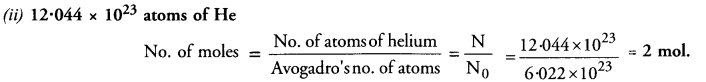Question 14.
Calculate the number of particles in each of the following :
(i) 46 g of sodium atoms
(ii) 8 g of oxygen (O2)
(iii) 0.1 mole of carbon atoms.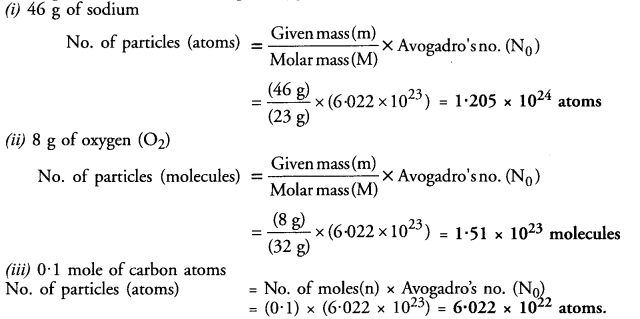Question 15.
If one mole of carbon weighs 12 grams, what is the mass (in gram) of one atom of carbon ?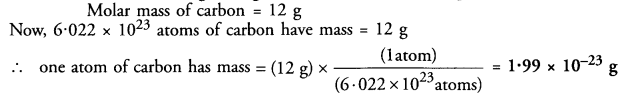Question 16.
Which has more number of atoms ?
(a) 100 grams of sodium
(b) 100 grams of iron
(Given : atomic mass of Na = 23 u ; Fe = 56 u)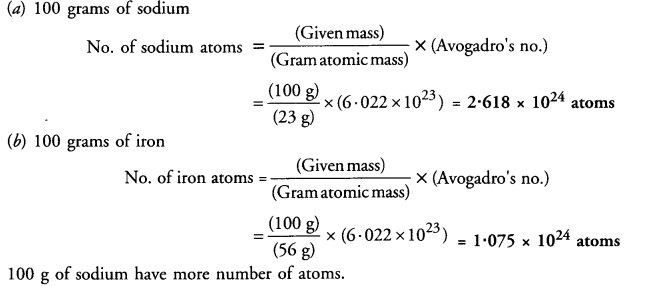NCERT END EXERCISE

Question 1.
0.24 g of sample of a compound of oxygen and boron was found by analysis to contain 0.096 g of boron
and 0.144 g of oxygen. Calculate the percentage composition of the compound by weight.Question 2.
When 3.0 g of carbon is burnt in 8.0 g of oxygen, 11.0 g of carbon dioxide is formed. What mass of carbon dioxide will be formed when 3.0 g of carbon is burnt in 50.0 g of oxygen ? Which law of chemical combination will govern your answer ? (CBSE 2011, 2012)
Carbon and oxygen react to form carbon dioxide according to the equation Carbon (C) + Oxygen (O2) > Carbon dioxide (CO2)In the first case :
3.0 g of carbon are burnt in 8.0 g of oxygen to form 11.0 g of CO2 In the second case :
3.0 g of carbon must also combine with 8.0 g of oxygen only. This means that (50 – 8) = 42 g of oxygen will remain unreacted.
The mass of CO2 in this case must be also 11 g.
The answer is based on Law of constant proportions.
In the second case, only 8.0 g of oxygen react although 50.0 g are available. This shows that the mass of carbon dioxide (11.0 g) formed depends upon the mass of carbon (3.0 g) or the substance present in smaller amount. In general, the substance (element or compound) present in smaller amount in a reaction limits the participation of the other reactants. It is quite often called limiting reactant. Carbon is the limiting reactant in this case. It limits the participation of oxygen and also the formation of carbon dioxide.

Question 3.
What are polyatomic ions ? Give examples. (CBSE 2015)
Polyatomic ions are the group of atoms which carry either positive charge (cations) or negative charge (anions). For example,

1. Carbonate ion (CO3)2-
2. Nitrate ion (NO3)
3. Ammonium ion (NH4)+
4. Phosphate ion (PO4)3-.

Question 4.
Write the chemical formulae of the following :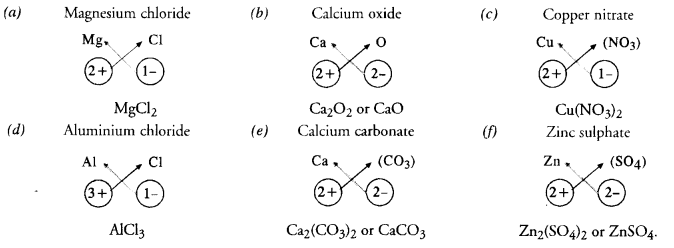Question 5.
Give the names of the elements present in the following compounds :
(a) Quick lime
(b) Hydrogen bromide
(c) Baking powder
(d) Potassium sulphate.
The names of elements present can be given only if the chemical formula of the compound is known. For example,
(a) Quick lime : It is the commercial name of the compound. Its chemical name is calcium oxide and the
chemical formula is CaO.
Elements present : calcium (Ca) : oxygen (O).
(b) Hydrogen bromide : The chemical formula of the compound is HBr
Elements present : hydrogen (H) ; bromine (Br).
(c) Baking powder : It is the commercial name of the compound. Its chemical name is sodium hydrogen carbonate and the chemical formula is NaHCO3
Elements present : sodium (Na), hydrogen (H), carbon (C), oxygen (O).
(d) Potassium sulphate : The chemical formula of the compound is K2SO4
Elements present : potassium (K), sulphur (S), oxygen (O).

Question 6.
Calculate the molar mass of the following substances :
(a) Ethyne, C2H2
(b) Sulphur molecule, S8
(c) Phosphorus molecule, P4 (Atomic mass of phosphorus = 31)
(d) Nitric acid, HNO3
(e) Hydrochloric acid, HCl.
(a) Ethyne, C2H2
Molar mass of C2H2 = (2 x Atomic mass of C) + (2 x Atomic mass of H)
= (2 x 12 u) + (2 x 1 u) = 26 u.
(b) Sulphur molecule, S8
Molar mass of S8 = 8 x Atomic mass of S = (8 x 32 u) = 256 u
(c) Phosphorus molecule, P4
Molar mass of P4 =4 x Atomic mass of P = (4 x 31 u) = 124 u
(d) Nitric acid, HNO3
Molar mass of HNO3 = (1 x Atomic mass of H) + (1 x Atomic mass of N) + ( 3 x Atomic mass of O)
= (1 x 1 u) + (1 x 14 u) + (3 x 16 u) = 63 u.
(e) Hydrochloric acid, HCl.
Molar mass of HCl = (1 x Atomic mass of H) + (1 x Atomic mass of Cl)
= (1 x 1 u) + (1 x 35.5 u) = 36.5 u.

Question 7.
What is the mass of :
(a) 1 mole of nitrogen atoms ?
(b) 4 moles of aluminium atoms (Atomic mass of aluminium = 27) ?
(c) 10 moles of sodium sulphite (Na2SO3) ?
(a) 1 mole of nitrogen atoms
Mass of 1 mole of nitrogen (N) atoms = 14 u
(b) 4 moles of aluminium atoms
Mass of 1 mole of aluminium (Al) atoms = 27 u
Mass of 4 moles of aluminium (Al) atoms = 4 x 27 = 108 u
(c) 10 moles of sodium sulphite (Na2SO3)
Molar mass of Na2SO3 = 2 x Atomic mass of Na + Atomic mass of S + 3 x Atomic mass of O
= 2 x 23 + 32 + 3 x 16 = 126 u
1 mole of sodium sulphite has mass = 126 u
10 moles of sodium sulphite have mass = 10 x 126 = 1260 u.

Question 8.
Convert into moles
(a) 12 g of oxygen gas
(b) 20 g of water
(c) 22 g of carbon dioxide.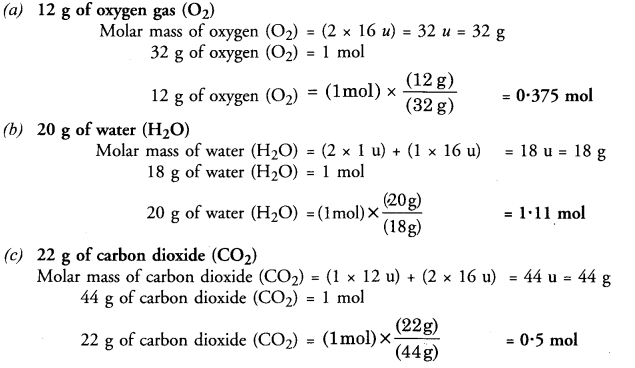Question 9.
What is the mass of :
(a) 0.2 mole of oxygen atoms ?
(b) 0.5 mole of water molecules ?
(a) 0.2 mole of oxygen atoms
Mass of 1 mole of oxygen (O) atoms = 16 u
Mass of 0.2 mole of oxygen (O) atoms = 0.2 x 16 = 3.2 u
(b) 0.5 mole of water molecules
Mass of 1 mole of water (H2O) molecules = 2 x 1 + 16 = 18 u
Mass of 0.5 mole of water (H2O) molecules = 0.5 x 18 = 9 u.

Question 10.
Calculate the number of molecules of sulphur (Sg) present in 16 g of solid sulphur.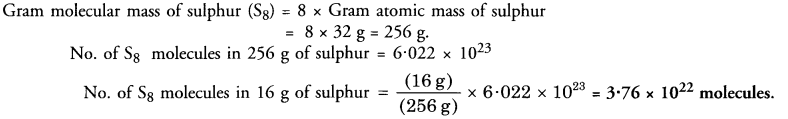Question 11.
Calculate the number of aluminium ions in 0.051 g of aluminium oxide (Al2O3). (CBSE 2012)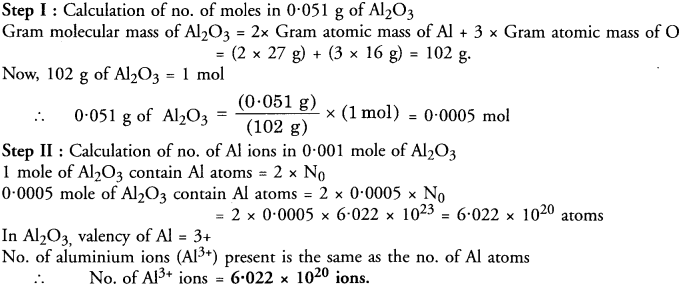Question 1.
Out of atoms and molecules, which can exist independently ?
Molecules can exist independently. However, the atoms of noble gases (He, Ne, Ar, Kr, Xe) can also exist independently.

Question 2.
What does the symbol ‘u’ represent ?
The symbol V represents unified mass.

Question 3.
Write the chemical symbols and Latin names of
(i) gold
(ii) mercury ?
(i) Au (Aurum)
(ii) Hg (Hydrargyrum).

Question 4.
Are the mass of a molecule of a substance and its molar mass same ?
No, they are different.

Question 5.
How are mass, molar mass and number of moles of a substance related to each other ?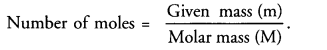Question 6.
Avogardo’s number represents how many particles ?
Avogadro’s number (Ng) represents 6.022 x 1023 particles.

Question 7.
Give an example of
(i) a divalent anion
(ii) a trivalent cation
(iii) a monovalent anion.
(i) (SO4)2-
(ii) Al3+
(iii) Cl

Question 8.
Calculate the molar mass of ethyl alcohol (C2H5OH).
Molar mass of C2H5OH= (2 x gram atomic mass of C) + (6 x gram atomic mass of H)
+ (1 x Atomic mass of O)
= (2 x 12 g) + (6 x 1 g) + (1 x 16 g) = 46 g.

Question 9.
If one mole of oxygen atoms weigh 16 grams, calculate the mass of one atom of oxygen (in grams).
(CBSE 2014)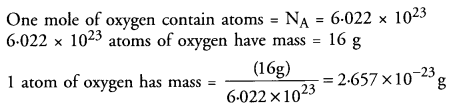Question 10.
What is the valency of calcium in CaCO3 ?
The valency of Ca in CaCO3 is 2+ (i.e. Ca2+).

Question 11.
What happens to an element ‘A’ if its atom gains two electrons ?
It changes to a divalent anion (A2-).

Question 12.
Why is a cation so named ?
When electric current is passed through the solution of a salt like sodium chloride (NaCl), the positive ion (Na+) migrates towards cathode (negative electrode). It is therefore, called cation. Please remember that
• Positive ion migrating towards cathode on passing electric current is known as cation.
• Negative ion migrating towards anode on passing electric current is known as anion.

Question 13.
An element Z forms an oxide with formula Z2O3. What is its valency ?
The valency of the element Z in Z2O3 is 3+.

Question 14.
The valency of an element A is 4. Write the formula of its oxide.
The formula of the oxide is A2O4 or AO2.

Question 15.
An element X has valency 3 while the element Y has valency 2. Write the formula of the compound between X and Y.
The formula of the compound between X and Y is X2Y3.

Question 16.
Formula of the carbonate of a metal M is M2CO3. Write the fomula of its chloride.
The valency of the metal (M) in M2CO3 is (1+) i.e. metal exists as M+ ion. Therefore, the formula of metal chloride is MCl.

Question 17.
What do you understand from the statement “relative atomic mass of sulphur is 32”. (CBSE 2012)
This means that an atom of sulphur is 32 times heavier as compared to 1/12 of the mass of 1 atom of C — 12(1 u).

Question 18.
Calculate the formula unit mass of CaCl2.
Formula unit mass of CaCl2 (Calcium chloride)
= (1 x Atomic mass of Ca) + (2 x Atomic mass of Cl)
= (1 x 40 u) + (2 x 35.5 u) = 111 u.

Question 19.
Which of the following represents the correct chemical formula ?
(a) NaSO4
(b) CaPO4
(c) ZnS
(d) AlSO4.
The formula (c) represents the correct formula. Both the ions are divalent i.e. Zn2+ and S2-. The name of the compound is zinc sulphide.

Question 20.
Sample A contains one gram molecules of oxygen molecules and sample B contains one mole of oxygen molecules. What is the ratio of the number of molecules in both the sample ?
One gram molecules and one gram mole contain the same number of molecules (6.022 x 1023). Therefore, the ratio is 1 : 1.

Question 21.
Gram molecular mass of ammonia (NH3) is 17 g. Is it correct to regard it as formula unit mass also ?
No, it is not correct. Ammonia exists in molecular form and is not an ionic compound made up of cation and anion. Therefore, it cannot have formula unit mass. It has only molecular mass.

Question 22.
Give one example each of polyatomic element and polyatomic ion.
Polyatomic element (P4) ; Polyatomic ion (SO42-).

Question 23.
Name the element which is used as a reference for the atomic masses of the elements.
1/12 of the mass of carbon atom taken as 12 u is used as a reference for the atomic masses of the elements.

Question 24.
Four samples of water [H2O] are collected from different sources. Each sample on analysis was found to contain same percentage of oxygen. Which law of chemical combination is demonstrated by the above observation ?
(CBSE 2014)
The law of constant combination is demonstrated by this observation.

Question 25.
List the elements present in
(i) quick lime
(ii) sodium hydrogen carbonate.
(i) The chemical name of quick lime is calcium oxide. Its chemical formula is CaO. Elements present are Ca and O.
(ii) The chemical formula of sodium hydrogen carbonate is NaHCO3. The elements present are Na, H, C and O.

Question 26.
Cenvert into moles :
(i) 20 g of water
(ii) 20 g of carbon dioxideQuestion 27.
(a) How many moles are present in 11.5 g of sodium ?
(b) The mass of an atom of element (X) is 2.0 x 10-23 g. Calculate its atomic mass.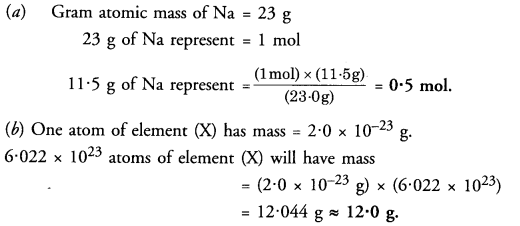Question 28.
(a) How many particles are represented by 0.25 mole of an element ?
(b) Out of 4 g of methane (CH4) and llg of CO2, which has more molecules ?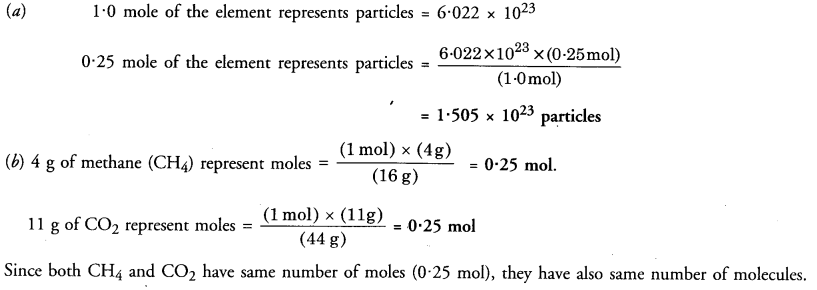Question 29.
What is the number of molecules present in 1.5 mole of ammonia (NH3) ?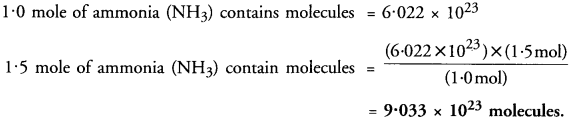Question 30.
Write the chemical symbols of two elements
(a) Which are formed from the first letter of the elements name.
(b) Whose name has been taken from the names of the elements in Latin.
(a) Boron (B), carbon (C)
(b) Argentum (Ag), Kalium (K).

Question 31.
(a) Four samples of carbon dioxide (CO2) were prepared by using different methods. Each sample on analysis was found to contain 27.27% carbon by mass. Name the law which is in agreement with this observation.
(b) Explain why the number of atoms in one mole of hydrogen gas is double the number of atoms in one mole of helium gas.
(a) Carbon dioxide consists of elements carbon and oxygen. Since the percentage of carbon in each sample is fixed, that of oxygen must be also fixed. This is according to law of constant proportions.
(b) Hydrogen gas is diatomic in nature (H2) while helium gas is monoatomic (He). As a result, the number of atoms in one mole of hydrogen. (2 x NA) are expected to be double as compared to number of atoms in one mole of helium (NA).

Question 32.
1022 atoms of an element ‘X’ are found to have a mass of 930 mg. Calculate the molar mass of the element ‘X’?Question 33.
(a) If the valency of carbon is 4 and that of sulphur is 2, write the formula of the compound formed between carbon and sulphur atoms. Also name the compound.
(b) What is wrong with the statement T mole of hydrogen ?
(a) The formula of compound can be written by exchanging the valencies (cross-over). Therefore, the expected formula is C2S4 or CS2. The compound is called carbon disulphide.
(b) The statement is not correct. We must always write whether hydrogen is in atomic form or molecular form. The correct statement is :

Question 34.
T mole of hydrogen atoms or one mole of hydrogen molecules.
Identify the cations and anions in the following compounds :
(a) CH3COONa
(b) NH3
(c) NH4Cl
(d) SrCl2.
(a) CH3COO, Na+
(b) It is a molecular compound
(c) NH4+ ,Cl (d) Sr2+, 2Cl

Question 35.
Classify the following based on atomicity
(a) O3
(b) P4
(c) S8. (CBSE 2012)
Atomicity is the number of atoms present in one molecule of an element. Based upon this, the atomicity of different molecules may be expressed as :
(a) 3
(b) 4
(c) 8.

Question 36.
Give the formulae of the compounds that will be formed from the following sets of elements.
(a) Calcium and fluorine
(b) Magnesium and oxygen
(c) Sodium and sulphur
(d) Carbon and chlorine
(e) Carbon and sulphur
(f) Nitrogen and hydrogen.
(a) CaF2
(b) MgO
(c) Na2S
(d) CCl4
(e) CS2
(f) NH3.

Question 37.
Which of the following symbols of elements are incorrect. Write correct symbols
(a) Iron (Ir)
(b) Gold (Au)
(c) Manganese (M)
(d) Potassium (Po)
(e) Zinc (ZN)
(f) Calcium (Ca).
(a) Iron (Fe)
(c) Manganese (Mn)
(d) Potassium (K)
(e) Zinc (Zn).

Question 38.
Verify by calculation that :
4 moles of CO2 and 6 moles of H2O do not have same mass in grams.
Molar mass of CO2 = 12 + 2 x 16 = 44 g
1 mole of CO2 has mass = 44 g
4 moles of CO2
have mass = 44 x 4 = 176 g
Molar mass of H2O = 2 x 1 + 16 =18 g
1 mole of H2O has mass = 18 g
6 moles of H2O have mass = 6 x 18 = 108 g.
This shows that these have different masses in grams.

Question 39.
A sample of vitamin C contains 2.48 x 1025 oxygen atoms. How many moles of oxygen atoms are present in the sample ?Question 40.
Calculate the total number of ions in 0.585 g of sodium chloride.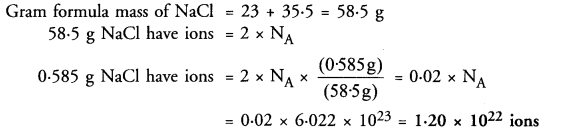Question 41.
A flask contains 4.4 g of CO2 gas. Calculate
(a) How many moles of CO2 gas does it contain ?
(b) How many molecules of CO2 gas are present in the sample.
(c) How many atoms of oxygen are present in the given sample.
(Atomic mass of C = 12 u. O = 16 u)Question 42.
Determine the molecular mass of :
(i) NH4OH
(ii) K2CO3
(iii) CH3COOH Given atomic masses :
H = 1.0 u ; O = 16.0 u ; C = 12.0 ; K = 39.0 u ; N = 14.0 u
(i) Molecular mass of NH4OH
= Atomic mass of N + 5 x (Atomic mass of H) + Atomic mass of O
= 14 + 5 x 1 + 16 = 14 + 5 + 16 = 35 u
(ii) Molecular mass of K2CO3
= 2 x (Atomic mass of K) + Atomic mass of C + 3 x (Atomic mass of O)
= 2 x 39 + 12 + 3 x 16 = 138 u
(iii) Molecular mass of CH3COOH
= 2 x (Atomic mass of C) + 4 x (Atomic mass of H) + 2 x (Atomic mass of O)
= 2 x 12 + 4 x 1+2 x 16 = 60 u

Question 43.
(a) What is Avogadro Constant ?
(b) Calculate the number of particles present in 56 g of N2 molecule.
(a) Avogadro’s number is the number of particles (atoms, ions, molecules etc.) present in one mole of any substance. It is denoted either as NA or as NQ. The number is also called Avogadro’s constant because its value is fixed (6.022 x 1023) irrespective of the nature of the particles.
(b) Molar mass of N2 = 14 x 2 = 28 g
28 g of N2 have molecules or particles = NA = 6.022 x 1023
56 g of N2 have molecules or particles = NA = 6.022 x 1023 x 2 = 1.204 x 1024

Question 44.
(a) An element ‘X’ exhibits variable valencies 3 and 5. Write the formulae of the chlorides of the element.
(b) What is the ratio by mass of the elements present in the chemical formula of magnesium oxide.
(a) The formulae of the chlorides of the element ‘X’ = XCl5 and XCl3.
(b) The chemical formula of magnesium oxide is MgO. The elements are present in the ratio of 24 : 16 or 3 : 2.

Question 45.
Give the example of trivalent cation and monovalent anion. Write the formula of the compound formed by their combination. .
Trivalent cation : Al3+ ; Monovalent anion : Cl
Formula of the compound : AlCl3.

Question 46.
(a) State the law of conservation of mass
(b) What mass of silver nitrate will react with 5.85 g of sodium chloride to produce 14.35 g of silver chloride and 8.5 g of sodium nitrate if the law of conservation of mass is true ?
(a) The total mass of the products in a physical change or a chemical reaction is equal to the total mass of the reactants that have combined. The law may also be stated in another form. The mass can neither he created nor destroyed in a physical change or a chemical reaction.
(b) The chemical reaction leading to products is :
Mass of reactants = x g + 5.85 g Mass of products = 14.35 g + 8.5 g According to law of conservation of mass
Mass of reactants = Mass of products . (x + 5.85) g = (14.35 + 8.5) g
x = (22.85 – 5.85) g = 17.0 g

Question 47.
(a) The mass of one molecule of a substance is 4.65 x 10-23 g. What is its molecular mass ? What could this substance be ?
(b) Which have more molecules? 10 g of sulphur dioxide (SO2) or 10 g of oxygen (O2) ?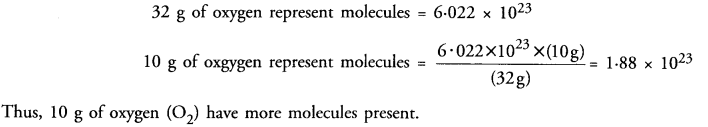Question 48.
Which has more atoms ?
(a) 10 g of nitrogen (N2)
(b) 10 g of ammonia (NH3)Question 49.
(a) Explain with the help of a labelled diagram an activity for the verification of law of conservation of mass.
(b) Find the number of atoms present in 100 g of sodium and 100 g of iron. (Given that Na = 23 u ; Fe = 56 u ;
(a) The total mass of the products in a physical change or a chemical reaction is equal to the total mass of the reactants that have combined. The law may also be stated in another form. The mass can neither he created nor destroyed in a physical change or a chemical reaction.
In other words, the mass remains unchanged or conserved in a chemical reaction. The law is also known as the Law of Indestructibility of Matter.
Explanation : Let us try to analyse as to what happens in a chemical reaction. To understand the same, let us consider a chemical reaction between barium chloride and sodium sulphate. When the solutions of these reactants prepared separately in water are mixed, the following chemical reaction takes place :
Barium chloride + Sodium sulphate———– > Barium sulphate + Sodium chloride (white precipitate)
If we look at this reaction, we find that the exchange of constituents has taken place between the reactants, i.e. chloride part of barium chloride has been exchanged by sulphate part of sodium sulphate. As such, no loss or gain in mass is expected. In other words, the mass remains conserved or does not change.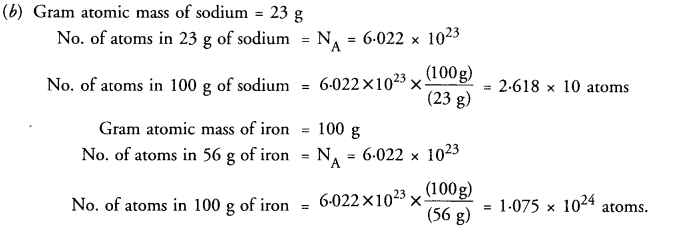Question 50.
(a) Give one point of difference between an atom and an ion.
(b) Give one example each of a polyatomic cation and a polyatomic anion.
(c) Identify the correct chemical name of FeSO4 from the given names Ferrous sulphate, Ferrous sulphide, Ferrous sulphite.
(d) Write the chemical formula for the chloride of magnesium.
(a) An atom is always neutral in the sense that it does not carry any charge. An ion carries either positive charge (cation) or negative charge (anion).
(b) Ammonium (NH4)+ ion is a polyatomic cation while sulphate (SO4)2- ion is a polyatomic anion.
(c) Correct chemical name of FeSO4 is ferrous sulphite.
(d) Chemical formula for the chloride of magnesium is MgCl2.

Question 51.
(a) What do the following observations stand for ?
(i) 2O
(ii) 3O2
(b) Which amongst the following has more number of atoms and how much ?
(i) 11.5 g of sodium
(ii) 15.0 g of calcium